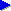Home# Introduction to binary numbers

As human beings– at least in most societies in the "developed" world– we tend to think of numbers in a system of tens. We generally represent numbers of any size using ten digits; every time we need a number that is a number of ten times greater (or ten times smaller after the decimal point), we tack on another digit. Historically, this "thinking in tens" probably came about because we have ten fingers. But it's essentially just a convention. We could actually base our numbering system on any other number of digits, instead of our conventional base 10 or decimal system.

For example, if we really wanted, we could use a base 7 numbering system. In this system, instead of having units, tens, hundreds etc, we would instead have units, sevens, forty-nines etc. Our decimal numbers 0-6 would still be 0-6 in base 7, but then our decimal number 7 would become 10 in the base 7 system, to represent "one seven plus zero units". The number 21 in base 7 would mean "two sevens plus one", or 15 in our usual system decimal. For humans used to decimal, using base 7 would seem like a crazy and inconvenient system, but theoretically it would work just as well1.

For all mainstream computers, the most "convenient"2 numbering system turns out to be binary or base 2. As you may have guessed, in this system there are just two digits: 0 and 1. You can also think of them respectively as "off" and "on", or "false" and "true". In this system, every digit to the left represents twice the value of the previous digit. In decimal, successive digits represent mulitples of 1, 10, 100, 1000 etc, multiplying by 10 each time. In binary, successive digits represent multiples of 1, 2, 4, 8, 16 etc, multiplying by 2 each time. The following illustration shows how the number 77 is represented in binary:Each box represents a successive binary digit. Just as in decimal, the digit representing the smallest magnitude appears on the right. Boxes coloured blue represent digits that are "on" or 1. So for each box that is "on", we add on that box's "value".

### Counting in binary

The table opposite illustrates how to represent the first few natural numbers in binary. Each number is shown as four figures of binary, so for the smaller numbers we have some leading zeroes. We'll see later that it's conventional in computing to represent binary numbers with some fixed number of digits suitable for our purposes, usually a multiple of 4 or 8. This is not really different to the decimal system, where occasionally (or at least for engineering convenience) it is the convention to use a fixed number of digits. For example, a web page counter might display a number such as 000637, but it means exactly the same as 637.

To reach a sum of zero in binary, we don't have to add any digits or "boxes" together, so the binary representation is all zeroes. For 1, we "set" the rightmost column, which represents the number of units. To represent decimal 2, we can't put a 2 in the units column, because we're only "allowed" to use the digits 0 and 1 in binary. So instead, we set a 1 in the second (rightmost) column, which represents the number of "2s". For decimal 3, we can now put a 1 in the units column; the binary 0011 thus means "1 + 2". Then for 4, we can't add anything to either the units or the 2s column, so instead we zero these columns and put a 1 in the "4s" column (remember, each time we go one column to the left, its value multiplies by 2), the third from the right. And so on.

Binary representation of the first few positive integers
DecimalBinary
00000
10001
20010
30011
40100
50101
60110
70111
81000
91001
101010

At this point, it's worth taking a moment to make sure you understand how binary works. On the next page, we'll look at the use of binary in computing.

1. There's actually a very subtle difference in the choice of numbering system which we'll return to later. The choice of base affects which fractions can be represented exactly with a fixed number of digits. For example, in decimal, it is impossible to represent "one seventh" exactly with a fixed number of digits after the decimal point: we end up with a recurring sequence "0.142857142857...". But in base 7, we could represent it exactly as "0.1".
2. Binary is convenient essentially for engineering reasons. Rather than having multiple voltages to represent different numbers, it's more reliable to measure whether a current is "present or not". Rather than having different proportions of a material magnetised in different directions, it's easier to detect whether a material is basically magnetised in "one direction or the other".